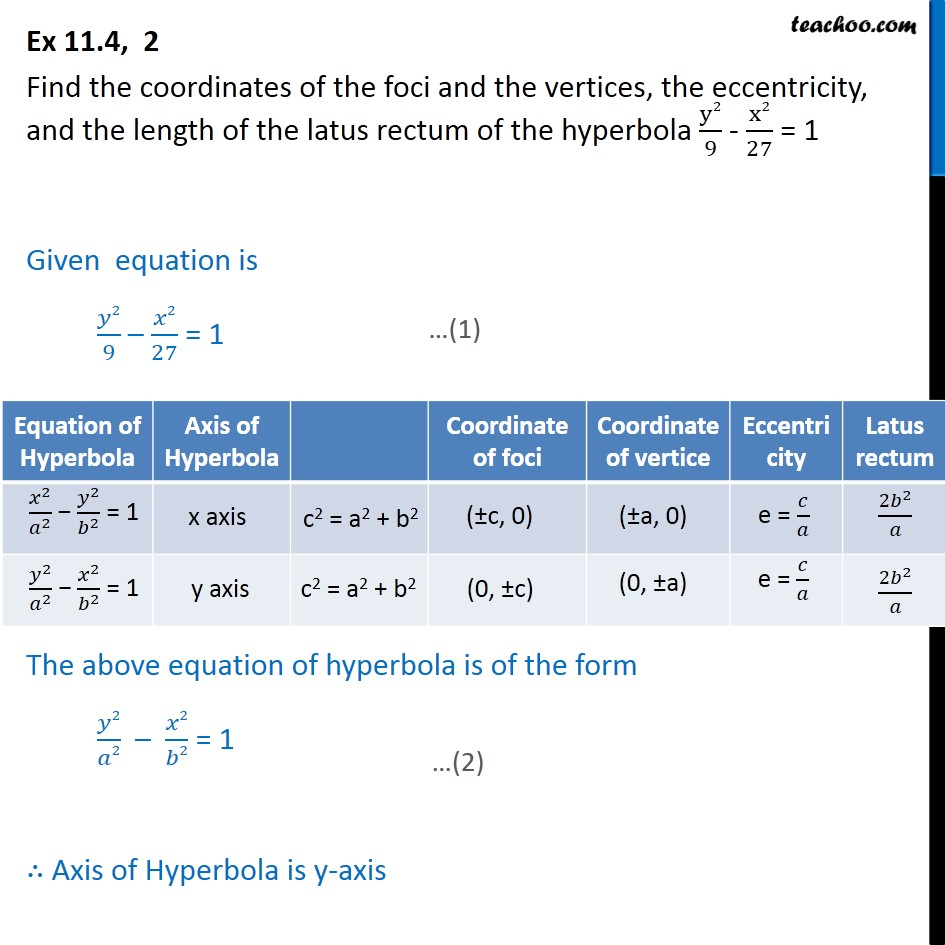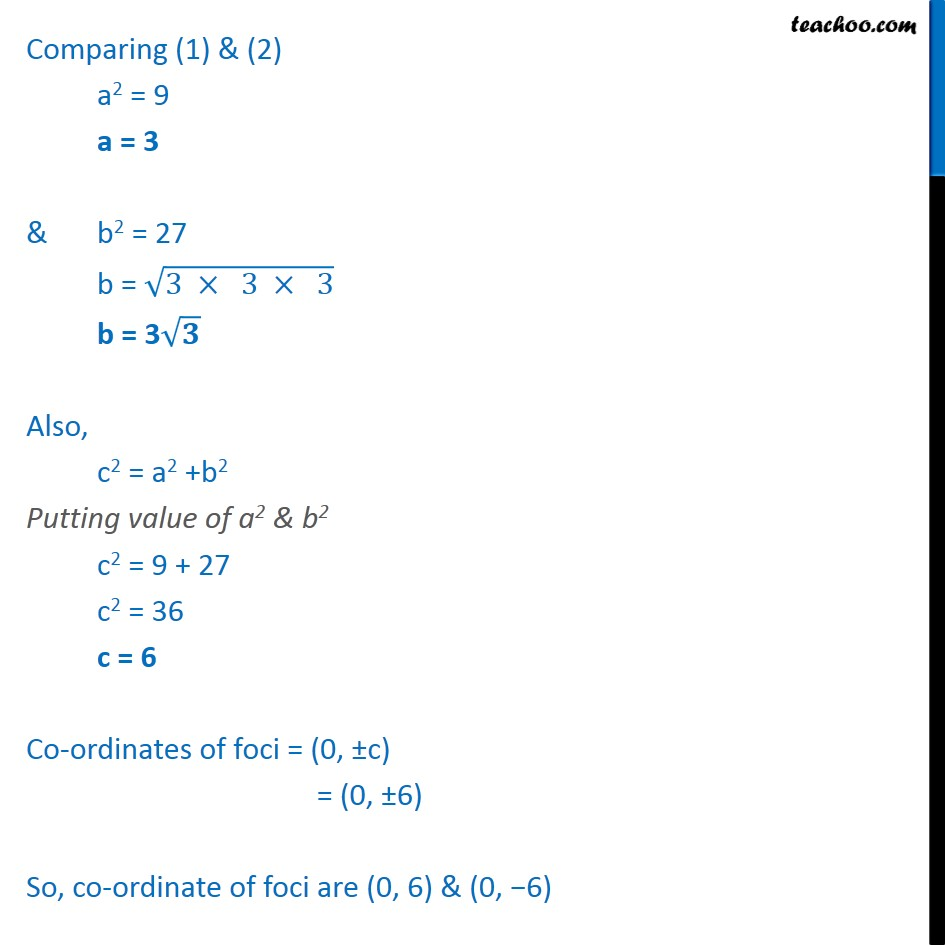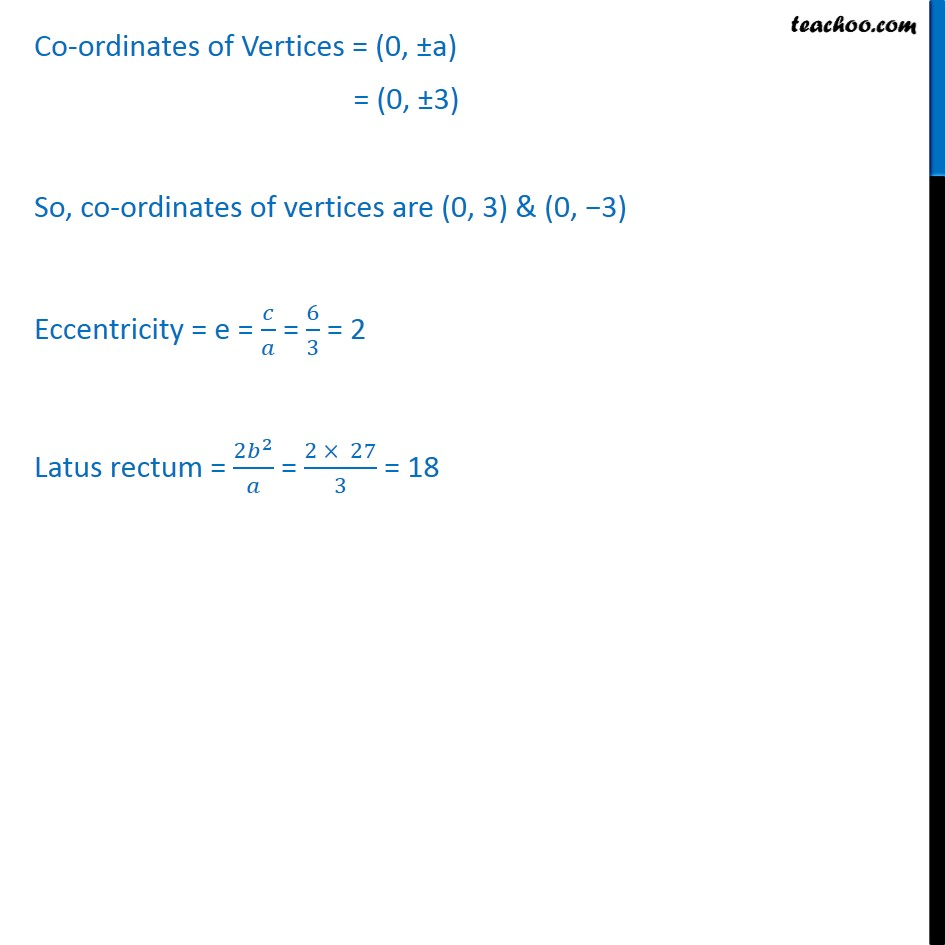Ex 11.4

Chapter 11 Class 11 Conic Sections
Serial order wiseGet live Maths 1-on-1 Classs - Class 6 to 12

### Transcript

Ex 11.4, 2 Find the coordinates of the foci and the vertices, the eccentricity, and the length of the latus rectum of the hyperbola ﷐y2﷮9﷯ - ﷐x2﷮27﷯ = 1 Given equation is ﷐𝑦2﷮9﷯ – ﷐𝑥2﷮27﷯ = 1 The above equation of hyperbola is of the form ﷐𝑦2﷮𝑎2﷯ – ﷐𝑥2﷮𝑏2﷯ = 1 ∴ Axis of Hyperbola is y-axis Comparing (1) & (2) a2 = 9 a = 3 & b2 = 27 b = ﷐﷮3 × 3 × 3﷯ b = 3﷐﷮𝟑﷯ Also, c2 = a2 +b2 Putting value of a2 & b2 c2 = 9 + 27 c2 = 36 c = 6 Co-ordinates of foci = (0, ±c) = (0, ±6) So, co-ordinate of foci are (0, 6) & (0, −6) Co-ordinates of Vertices = (0, ±a) = (0, ±3) So, co-ordinates of vertices are (0, 3) & (0, −3) Eccentricity = e = ﷐𝑐﷮𝑎﷯ = ﷐6﷮3﷯ = 2 Latus rectum = ﷐2﷐𝑏﷮2﷯﷮𝑎﷯ = ﷐2 × 27﷮3﷯ = 18